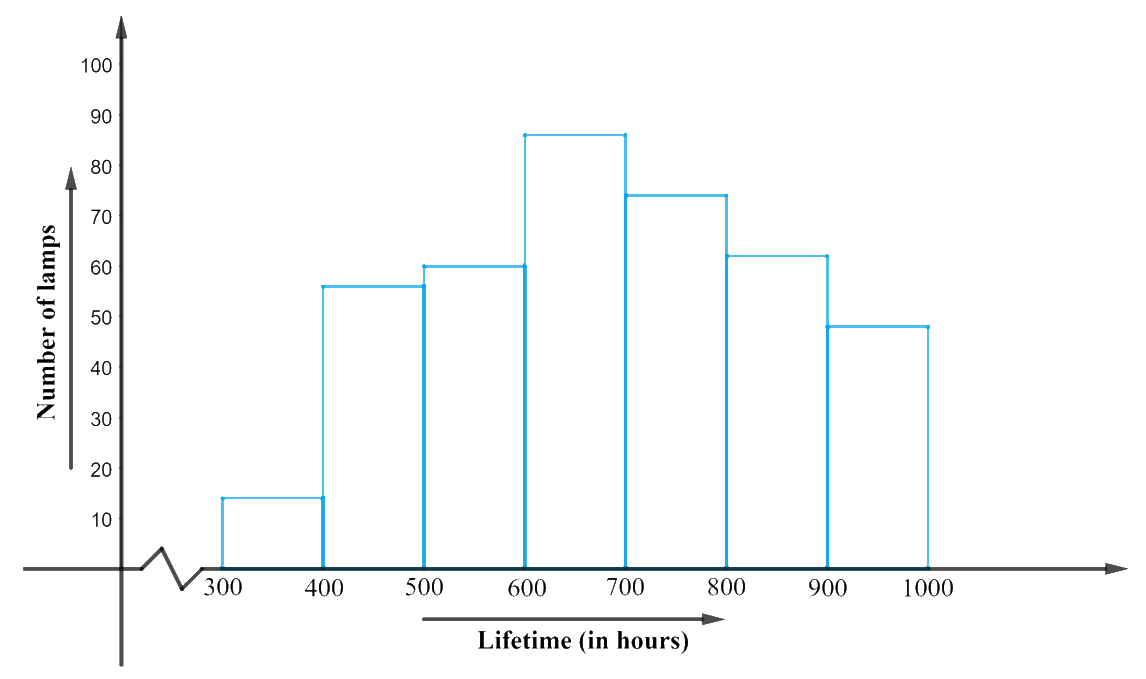# Ex.14.3 Q5 Statistics Solution - NCERT Maths Class 9

## Question

The following table gives the life times of $$400$$ neon lamps:

 Life time (in hours) Number of lamps $$300 - 400$$ $$14$$ $$400 - 500$$ $$56$$ $$500 - 600$$ $$60$$ $$600 - 700$$ $$86$$ $$700 - 800$$ $$74$$ $$800 - 900$$ $$62$$ $$900 - 1000$$ $$48$$

(i) Represent the given information with the help of a histogram.

(ii) How many lamps have a life time of more than $$700$$ hours?

Video Solution
Statistics
Ex exercise-14-3 | Question 5

## Text Solution

What is known?

Life times of $$400$$ neon lamps.

What is Unknown?

(i) A histogram representation for the given data.

(ii) Number of lamps that have a lifetime of more than $$700$$ hours.

Reasoning:

The given data can be represented with the help of a histogram as above:

1. Represent the ‘lifetime (in hours) in $$x$$-axis.
2. Represent the ‘number of lamps’ in $$y$$-axis.
3. Class intervals are continuous.
4. Take “$$1\,\rm unit = 10\,\rm lamps$$” on $$y$$-axis as the lowest value of frequency is $$14$$ and highest $$86.$$
5. Also since the first interval is starting from $$300$$ and not ‘$$0$$’, we show it by marking a ‘kink’ or a break on the $$x$$-axis.

Steps:From the above graph, it can be concluded that: The number of neon lamps having their lifetime more than $$700$$ is under class intervals “$$700 – 800, 800 – 900, 900 – 1000$$”.

Hence, their corresponding frequencies when added up will be $$(72 + 62 + 48) 184$$ lamps.

Learn from the best math teachers and top your exams

• Live one on one classroom and doubt clearing
• Practice worksheets in and after class for conceptual clarity
• Personalized curriculum to keep up with school